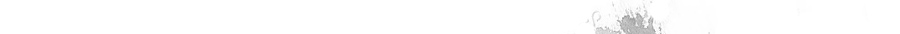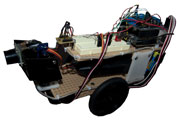# Robot 1

The Robot 1 is designed to drive in the direction with the most room to travel. (I know, please hold your excitement in).

The Robot 1 is the first of my attempts to learn the Arduino.I've used a number of prebuilt modules to send real world data back to the controlling device (and they connect pretty easily). Modules tend to be between \$10-\$30 AUD and are worth every cent for a novice.

It's far from perfect, but it was certainly a good place to start.

The following is the sketch uploaded directly to the Arduino.

``` #include <AFMotor.h> #include <Servo.h>   // Define Servo Wheels Servo servoLeft; Servo servoRight;   // Define Ping Sensor Chassis Serco Motors (the two servos used to make the ping sensor look left/right and up/down) Servo servoUpDown; Servo servoLeftRight;   // Setup AM3 Accellerometer (used to idnetify the tilt of the robot) int xAxis = A2; int axisValue = 0; int gravity;   // Setup Ping Sensor int distSensor = 0; const int pwPin = 8; long pulse, inches, cm; // Setup Hall Effect Sensor - i.e Wheel monitor const int hallPin = 7;     void setup(){   int i = 0;   // Intitiate Serial connection for debugging   Serial.begin(9600);   // attach servo's to Pins and stop - Note: Motor shield uses Pin 9,10   servoUpDown.attach(3);   servoLeftRight.attach(4);   servoLeft.attach(10);   servoRight.attach(9);   servoLeft.write(95); // 1 to 94 Backward, 96 to 200 Forward   servoRight.write(95); // 1 to 94 Forward, 96 to 200 Backward   // attach Hall Effewt Sensor   pinMode(hallPin, INPUT);   // Set sensor motors to front   servoLeftRight.write(86);   servoUpDown.write(1);   // attach Ping Sensor   pinMode(pwPin, INPUT);   // set gravity level   // average out reads to accommodate misreads and fluctuation   for (i=0; i<10; i++) {     axisValue = analogRead(xAxis);     gravity += axisValue;     delay(10);   }   gravity /= 10;   // Give user 5 seconds before robot starts   delay(5000); } // *********************************   void loop(){   // Move forward   servoLeft.write(200); // 1 to 94 Backward, 96 to 200 Forward   servoRight.write(83); // 1 to 94 Forward, 96 to 200 Backward   // Check Distance   checkDistance();   // if distance is < 30cm then check the direction to move in   if (distSensor < 30) {checkDirection();}   // Check vertical angle   adjustPingVertical(); }   // *********************************   void checkDirection(){   int leftDistance = 0;   int rightDistance = 0;   stopBot();   // Check Left   servoLeftRight.write(0);   delay(1500);   checkDistance();   leftDistance = distSensor;   // Check Right   servoLeftRight.write(180);   delay(2500);   checkDistance();   rightDistance = distSensor;   // set Ping Sensor back to forward facing   servoLeftRight.write(86);   delay(2000);   // Define direction   if (leftDistance < rightDistance && rightDistance > 30){     goRight();   }   else if (leftDistance > rightDistance && leftDistance > 30){     goLeft();   }   else {     turnAround();   } }   // *********************************   void checkDistance(){   int i = 0;   //Following code modified from original code by Author: Bruce Allen - 23/07/09   //Used to read in the pulse that is being sent by the MaxSonar device.   //Pulse Width representation with a scale factor of 147 uS per Inch.   // Average 8 readings to accomodate noise and error   for (i=0; i<8; i++) {     pulse = pulseIn(pwPin, HIGH);     //147uS per inch     inches = pulse/147;     //change inches to centimetres     cm = inches * 2.54;     distSensor += cm;     delay(50);   }   distSensor /= 8; }   // *********************************   void adjustPingVertical(){   int angle = 0;   int upDownAngle = 0;   int i = 0;   // read the angle - 400 is flat   axisValue = analogRead(xAxis);   // average out reads to accommodate misreads and fluctuation - divde by 2 to   accommodate angle   for (i=0; i<8; i++) {     angle = 400 - axisValue;     angle /= 2;     upDownAngle += angle;     delay(10);   }      upDownAngle /= 8;   upDownAngle = 1 + upDownAngle;     // Don't tilt the sensor greater than 90 degrees or below 0   if (upDownAngle > 90){upDownAngle = 90;}   if (upDownAngle < 1){upDownAngle = 1;}   servoUpDown.write(upDownAngle); } // *********************************   void goRight(){     int i = 0;   int hallState = 0;   hallState = digitalRead(hallPin);   // Set the wheels to turn the robot right   servoLeft.write(200); // 1 to 94 Backward, 96 to 200 Forward   servoRight.write(200); // 1 to 94 Forward, 96 to 200 Backward   // If wheel is currently on a magnet, move off magnet   while ( hallState == LOW){    hallState = digitalRead(hallPin);   } // end while   // count 2 magnets on the wheel   while (i != 2){     hallState = digitalRead(hallPin);     if (hallState == LOW){ // i.e on a magnet        i = i + 1;        while ( hallState == LOW){ // move off magnet          hallState = digitalRead(hallPin);        } // end while     } // end if   } // end while } // end goRight   // *********************************   void goLeft(){   int i = 0;   int hallState = 0;   hallState = digitalRead(hallPin);   // Set the wheels to turn right   servoLeft.write(1); // 1 to 94 Backward, 96 to 200 Forward   servoRight.write(1); // 1 to 94 Forward, 96 to 200 Backward   // move off magnet   while ( hallState == LOW){      hallState = digitalRead(hallPin);   } // end while   // count 2 magnets on the wheel   while (i != 2){     hallState = digitalRead(hallPin);     if (hallState == LOW){       i = i + 1;       while ( hallState == LOW){         hallState = digitalRead(hallPin);       } // end while    } // end if   } // end while } // end goLeft   // *********************************   void turnAround(){     int i = 0;   int hallState = 0;   hallState = digitalRead(hallPin);   // Set the wheels to turn right   servoLeft.write(1); // 1 to 94 Backward, 96 to 200 Forward   servoRight.write(1); // 1 to 94 Forward, 96 to 200 Backward   // move off magnet    while ( hallState == LOW){      hallState = digitalRead(hallPin);    } // end while   // count 2 magnets on the wheel   while (i != 4){     hallState = digitalRead(hallPin);     if (hallState == LOW){       i = i + 1;       while ( hallState == LOW){          hallState = digitalRead(hallPin);       } // end while     } // end if   } // end while   } // end turnAround   // *********************************   void stopBot(){     int hallState = 0;   hallState = digitalRead(hallPin);      // Stop when at magnet   while (hallState == HIGH){     hallState = digitalRead(hallPin);   }     // Stop Servos   servoLeft.write(95); // 1 to 94 Backward, 96 to 200 Forward   servoRight.write(95); // 1 to 94 Forward, 96 to 200 Backward }   ```

It could probably use a set of whiskers on the front for collision detection as the ping sensor isn't completely reliable on it's own. If you're on an angle, the ping can bounce off in another direction and not report the fact that it is about to hit a wall. You could also daisy chain up a few ping / ultrasonic sensors on various angles to alleviate the issue.